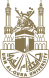# Umm Al-Qura University- 2016/06/09

Cumulative Rating Calculating

First: calculating the points of each course

Course is defined as a subject of the accredited study plan for each specialty during the semester. It is categorized by a number, symbol, title and description differentiating them from their equivalents in terms of content and level.

Points of each course recorded in the student’s timetable are calculated by computing the course grade multiplied by the course credit hours.

Second: Calculating the Semester Rating

The semester rating is calculated by the total of the semester course points divided by the total of the credit hours of the semester courses.

Third: Calculating the Cumulative Rating

The cumulative rating is calculated by the total of all the course points divided by the total of the course credit hours